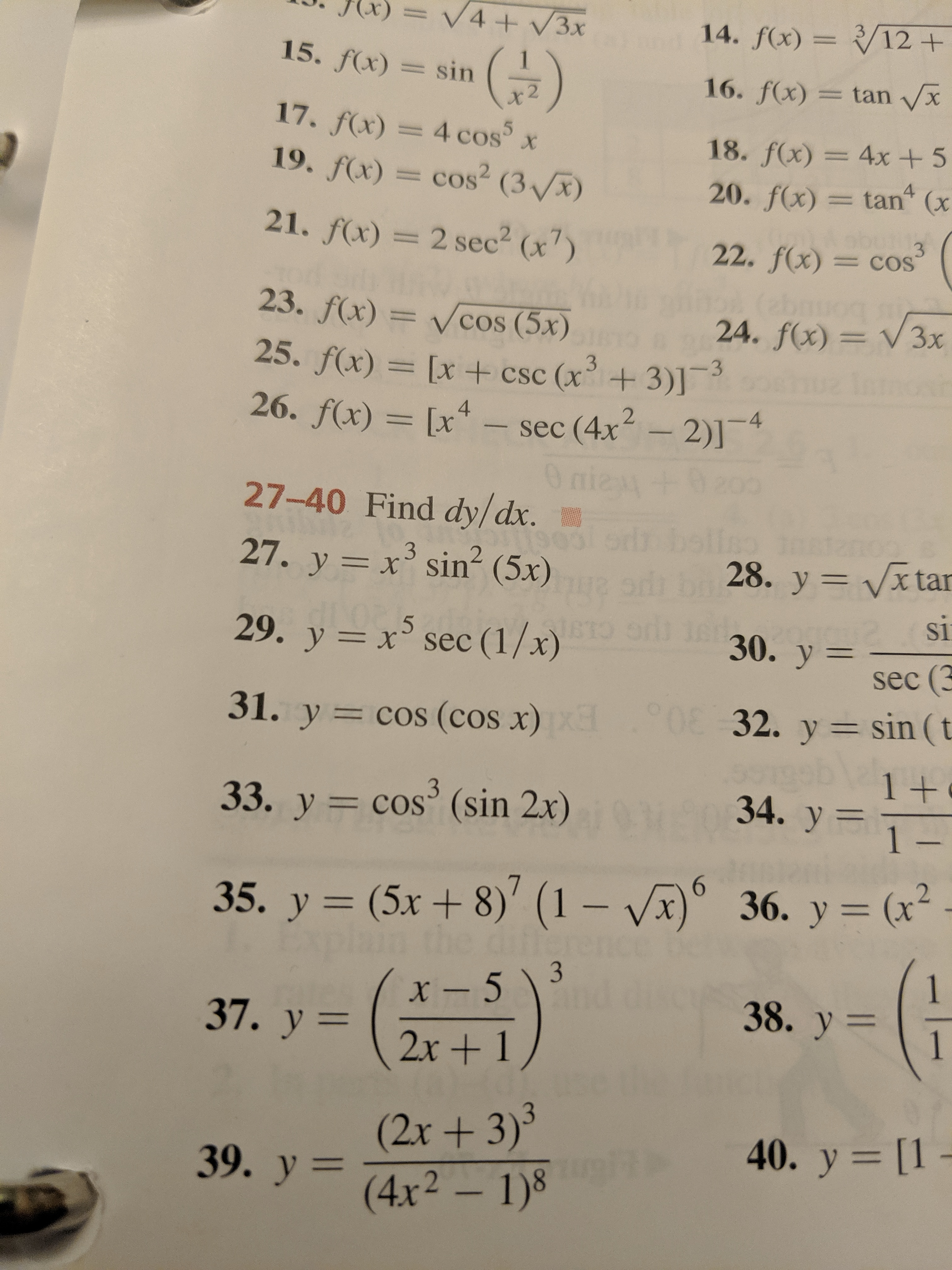# V4+/3x14. f(x)=/12 +15. f(x)sin16. f(x)= tan xx 2fx) = 4 cos5x.f(x) = cos2 ( 318. f(x)= 4x + 5tan (x420. f(x)01 121. f(x)= 2 sec2 (x)322. f(x)=COSabr24. f(x) V3x23. f(x)=Vcos (5x)25. f(x) = [x +csc (x+3)]26. f(x) [xsec (4x 2)]4O nie +227-40 Find dy/dx.27. y x sin2 (5x)28. y Vx tarXsi30. y=.529. y x sec (1/x)sec (3032. y sin (t31. y cos (cos x)1 +34. y 1-333. y = cos (sin 2x)636. у 3 (х*36. y= (x(5x + 8)' (1 - Vx35. y1x-51338. y37. у —2x+1(2x+ 3)339. y4x2 1)40. y [1 -

Question
13 views

#33.

y=cos3(sin2x)help_outlineImage TranscriptioncloseV4+/3x 14. f(x)=/12 + 15. f(x) sin 16. f(x)= tan x x 2 fx) = 4 cos5x .f(x) = cos2 ( 3 18. f(x)= 4x + 5 tan (x 4 20. f(x) 01 1 21. f(x) = 2 sec2 (x) 3 22. f(x) =COS abr 24. f(x) V3x 23. f(x)= Vcos (5x) 25. f(x) = [x +csc (x+3)] 26. f(x) [x sec (4x 2)]4 O nie + 2 27-40 Find dy/dx. 27. y x sin2 (5x) 28. y Vx tar X si 30. y= .5 29. y x sec (1/x) sec (3 032. y sin (t 31. y cos (cos x) 1 + 34. y 1- 3 33. y = cos (sin 2x) 6 36. у 3 (х* 36. y= (x (5x + 8)' (1 - Vx 35. y 1 x-513 38. y 37. у — 2x+1 (2x+ 3)3 39. y4x2 1) 40. y [1 - fullscreen
check_circle

Step 1

In order to compute the derivative of a given function, first differentiate the power te...

### Want to see the full answer?

See Solution

#### Want to see this answer and more?

Solutions are written by subject experts who are available 24/7. Questions are typically answered within 1 hour.*

See Solution
*Response times may vary by subject and question.
Tagged in

### Derivative